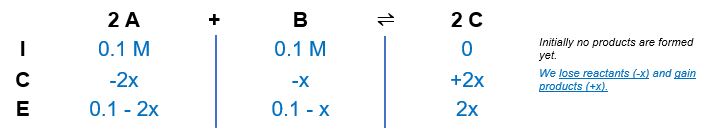# Problem: The following reaction has a Kc at 520. K equal to 1.50 × 10–8:2 A(aq) + B(aq) ⇌ 2 C(aq)If the reaction is started with 0.100 M A and 0.100 M B, what is the equilibrium concentration of C?A. 3.75 × 10–12 M B. 1.94 × 10–6 M C. 3.87 × 10–6 MD. 7.50 × 10–12 M E. 1.50 × 10–11 M

###### FREE Expert Solution

We’re being asked to determine the equilibrium concentrationof C given the following equilibrium reaction:

2 A(aq) + B(aq) ⇌ 2 C(aq)Kc = 1.5x10-8

We know that the initial amount of A and B is 0.1 M. From this, we can construct an ICE table:Kc is the equilibrium expression of the concentrations in the reaction. The general form of an equilibrium expression is:

96% (256 ratings)###### Problem Details

The following reaction has a Kc at 520. K equal to 1.50 × 10–8:

2 A(aq) + B(aq) ⇌ 2 C(aq)

If the reaction is started with 0.100 M A and 0.100 M B, what is the equilibrium concentration of C?

A. 3.75 × 10–12

B. 1.94 × 10–6

C. 3.87 × 10–6 M

D. 7.50 × 10–12

E. 1.50 × 10–11 M